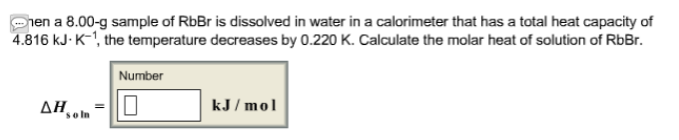# Problem: When a 8.00-g sample of RbBr is dissolved in water in a calorimeter that has a total heat capacity of 4.816 kJ • K-1, the temperature decreases by 0.220 K. Calculate the molar heat of solution of RbBr.

###### FREE Expert Solution
85% (408 ratings)###### Problem Details

When a 8.00-g sample of RbBr is dissolved in water in a calorimeter that has a total heat capacity of 4.816 kJ • K-1, the temperature decreases by 0.220 K. Calculate the molar heat of solution of RbBr.